# Using the "Quick Graph" functions

May, 2001

## Introduction

If you have the need for quick visualization of your simple field type data, we have provided a Python script, graph.py which includes several 2-D displays in simple formats: This page describes each of these in detail. There are several common keyword parameters for all these functions:
• width= specify the width of the window
• height= specify the height of the window
• panel= specify a Java JPanel to use; if omitted, these will all
Note that prior to using any of the methods from the graph.py script, you must either:
```import graph
```
or you must:
```from graph import *
```
If you choose the first form, the all your references to methods in must include "graph" -- that is: graph.histogram(data). If you use the second form then you omit the "graph" -- that is: histogram(data).

You may also have to import other things. For example, to use the Java Color, you will have to:

```from java.awt import Color
```
for example.

If you want, you can look at the Jython code for graph.py.

## Image

A simple display of a gray-scale image. This code:
```a=load("../data/mcidas/AREA0001")
graph.image(a)
```
produces this display: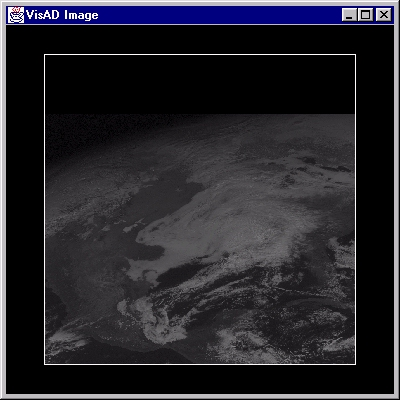## Scatter Plot

A scatter diagram, where the range values of one parameter are plotted against the range values for another.
```a=load("../data/mcidas/AREA0008")
b = extract(a,0)
c = extract(a,1)
graph.scatter(b,c)
```
produces this display: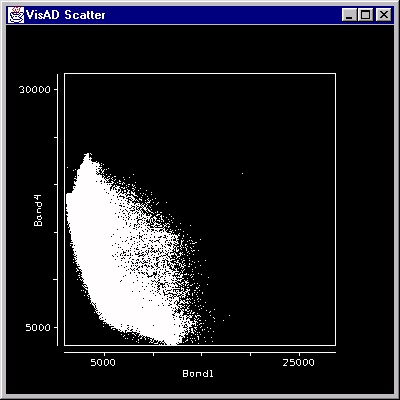## Histogram

A simple traditional "bar-graph" style of a histogram.
```a=load("../data/mcidas/AREA0001")
graph.histogram(a, 40, color=Color.cyan)
```
produces this display: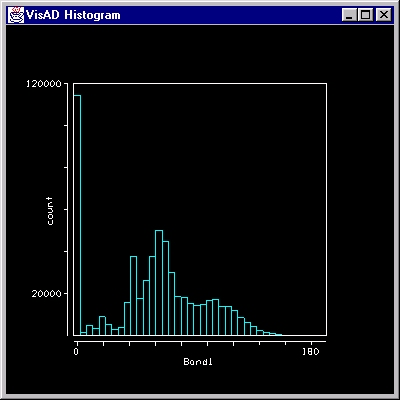## Line Plot

A simple single line plot.
```a=field( (1,2,3,1,2,3,1,2,3,1,2,3))
graph.lineplot(a, color=Color.orange)
```
produces this display: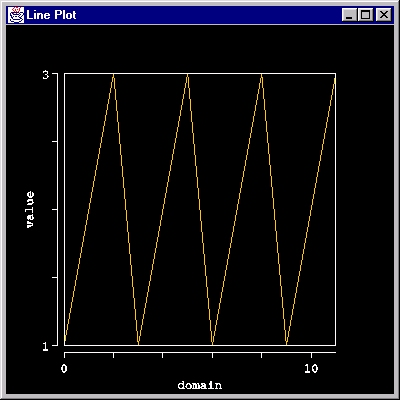## Contour

A simple display of iso-contours. This code:
```a=load("../data/text/example1.txt")
graph.contour(a)
```
produces this display: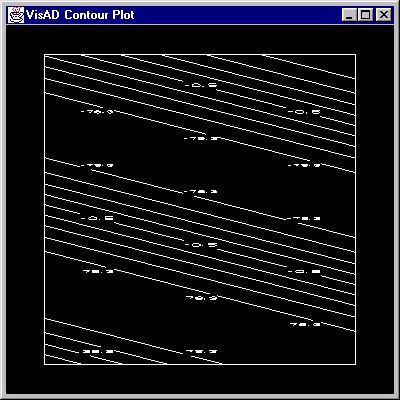## Animation

A simple display of an animation of images, including the VisAD Animation Widget. This code:
```a=load("../examples/images.nc")
graph.animation(a)
```
produces this display: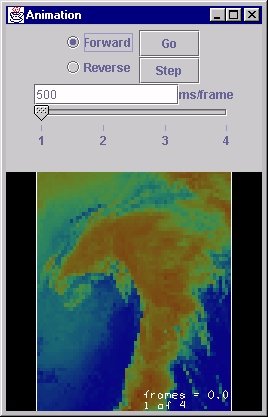You can also just use a sequence of JPEG or GIF images. For example:

```a=load("image0.jpg")